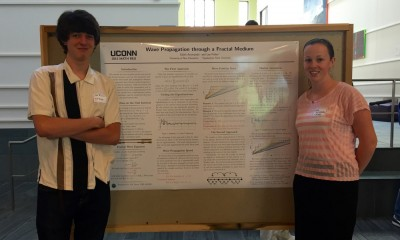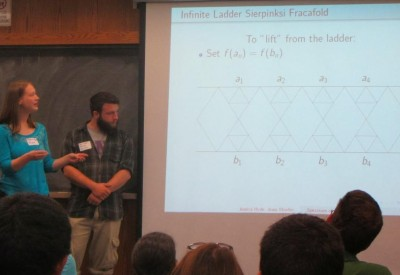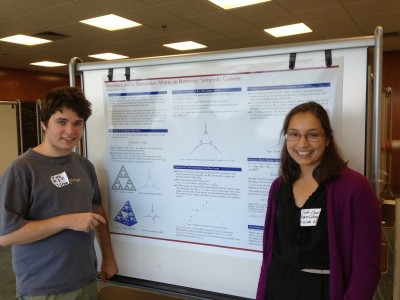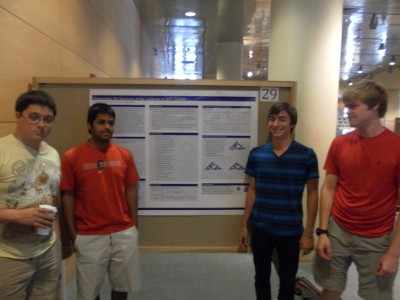# Spectral Analysis on Graphs Related to the Basilica Julia Set

July 28, 2017

## Overview

We analyze the spectra of a sequence of graphs constructed from the Schreier graphs of the Basilica group.  Our analysis differs from earlier work of Grigorchuk and Zuk in that it is based on a macroscopic decomposition of the graphs. This method gives precise information about the multiplicities of eigenvalues and, consequently, good information about the spectral measures of large graphs. It also permits a proof of the existence of gaps in the spectrum of limiting graphs.

# Spectrum of the Magnetic Laplacian on the Diamond Fractal

May 22, 2016

## Overview

The diamond fractal is a fractal that is obtained in the following manner.  Start with a graph with two vertices and an edge and replace the edge with two new vertices connected to our original vertices to obtain a diamond shaped graph.   The diamond fractal is defined to be the limiting object after continuing with the edge replacement indefinitely.  In the project, the spectrum of magnetic Laplacian operators on graph approximations to the diamond fractal was computed.

Given a level n approximation to the fractal with known magnetic field strengths through cells and holes, it is possible to determine the net magnetic field through the cells and holes of the preceding graph approximations.  The spectral similarity relation between the operators on successive graph approximations was worked out, with the corresponding spectral decimation polynomial depending on the magnetic field strengths.  A poster and talk on this work was presented at the REU Mini-Symposium at UConn.

# arXiv:1704.01609

## Presentation

Magnetic Spectral Decimation

Poster

# Power Dissipation in Fractal AC Circuits

May 21, 2016

## Supervisors

Alexander TeplyaevLuke Rogers, Antoni Brzoska, Ulysses Andrews

## Overview

In this project, Feynman’s analysis of an infinite ladder circuit is extended to fractal circuits, in particular, a Sierpinski Ladder circuit and two variants of a Hanoi circuit.   These circuits are constructed by taking the limit of graph approximations with inductors and capacitors placed along edges in a well-defined manner.  Inductors, capacitors and resistors all contribute to the impedance within a circuit; but whereas a resistor imparts a real impedance, inductors and capacitors impart a purely imaginary impedance.

For each circuit, the following was accomplished.  First, the net impedance between certain boundary points of the circuit was computed.  Second, the filter conditions for each circuit were found.  A circuit becomes a filter when the net impedance between boundary vertices has a positive real part!  Third, it was proved that these impedances can be obtained by placing a small positive resistance epsilon on each edge of the graph approximations, finding the limiting impedance between the boundary vertices, and then taking epsilon to zero.  Finally, the construction of harmonic functions on these circuits was outlined.

# arXiv:1605.03890

## Presentation

Fractal AC Circuits

Poster

May 2, 2016

# Group Members

Edith Aromando, Lee Fisher## Overview

We consider the wave equation on the unit interval with fractal measure, and use two numerical models to study wave speed and propagation distance.  The first approach uses a Fourier series of eigenfunctions of the fractal Laplacian, while the second uses a Markov chain to model the transmission and reflection of classical waves on an approximation of the fractal. These models have complementary advantages and limitations, and we conjecture that they approximate the same fractal wave.

Poster

# Spectrum of the Magnetic Laplacian on the Sierpinski Gasket

April 21, 2016## Overview

One project investigated magnetic gauge fields on the Sierpinski Gasket. After numerical experimentation, using Mathematica and MatLab, this team determined that specific portions of the spectrum of the Laplacian are unaffected by a perturbating magnetic field given by a harmonic 1-form of finite topological type and continued on to prove that this. In a specific case they also gave a description of the spectrum via a covering space and symmetry argument. They presented a poster and a talk on this work at the REU mini-conference at the University of Massachusetts, Amherst and gave a talk at the Young Mathematician’s Conference at Ohio State University.

## Presentation

Spectrum of the Magnetic Laplacian

arXiv.org

# Measurable Riemannian Structure on Higher Dimensional Sierpinski Gaskets## Overview

This team worked on the existence of measurable Riemannian structures in the sense of Kigami on higher dimensional Sierpinski-type gaskets. Some time ago, Kusuoka proved existence of a measure, metric (in the Riemannian sense) and gradient operator on a class of fractals that includes these gaskets, such that these objects bear the same relation to the Dirichlet form as do the Riemannian volume, metric and gradient on Euclidean space. Kigami later completed this picture in the case of the usual 3-vertex Sierpinski gasket by constructing a geodesic length that is the analogue of that occuring in the Riemannian case and proving Gaussian heat kernel estimates, and Kajino has subsequently proved very re ned estimates for the heat kernel in this setting. It was believed that although Kigami’s approach relied on certain 2-dimensional techniques the results would also be valid on Sierpinski-type gaskets with more vertices, and that is what our research team have proved. They presented a poster on this work at the REU mini-conference at the University of Massachusetts, Amherst, and are writing the results up for publication.

# arXiv:1703.03380

## Presentation

Geodesics and a Riemannian Metric on Harmonic Sierpinski Gaskets

# Determining the Spectrum of the Laplacian on 3N-Gaskets## Overview

The Laplacian is the central object of analysis on fractals. While most work on the Laplacian has been focused on computing the Laplacian spectrum on specific fractals, this group instead found the spectrum on an entire class of fractals—the 3N-Gaskets. This is the class of finitely ramified fractal 3N-Gons that are the attractors of iterated function systems containing 3N contraction mappings. For example, the 3-Gasket is the Sierpinski triangle.

The Laplacian on the fractal, and thus its eigenvalues, must be studied by examining the graph Laplacian on approximating graphs. The group found a function R(z) relating the Laplacian eigenvalues on consecutive graph approximations. They used R(z) along with the eigenvalues of the Laplacian on graph approximations to derive part of the spectrum on the next level. The rest of the spectrum was found using an “exceptional set”— a set of values which the group provided for arbitrary N. By repeating this infinitely many times, the spectrum of the Laplacian on the fractal could be found.

arXiv.org

# Analyzing Properties of the C. Elegans Neural Network: Mathematically Modeling a Biological System## Overview

The brain is one of the most studied and highly complex systems in the biological world. This group analyzed the brain of the nematode Caenorhabditis elegans. They used eigenvalues and eigenvectors of the Laplacian matrix — a matrix representation — of the neural network of the nematode brain with an eye on indicators of self-similarity.

The following is a list of the programs this group used in their research. Most of them were written in Matlab.

# The Strichartz Hexacarpet and Higher Dimensional Analogues## Overview

The Strichartz hexacarpet is a fractal which can be obtained by repeated barycentric subdivisions of a triangle. This group examined properties of graph approximations and looked at analogous fractals obtained by subdivisions of higher dimensional triangles — n-simplexes. They studied properties of random walks on these fractals, obtaining heat kernel estimates and resistance factors. See also Random Walks on Barycentric Subdivisions and the Strichartz Hexacarpet Experiment. Math. Volume 21, Issue 4 (2012), 402-417.

## Presentation

Random Walks on Barycentric Subdivisions and the Strichartz Hexacarpet

# Quantum Mechanics on Laakso Spaces

April 20, 2016

## Overview

This group first reviewed the spectrum of the Laplacian operator on a general Laakso space before considering modified Hamiltonians for the infinite square well, parabola, and Coulomb potentials. Additionally, they computed the spectrum for the Laplacian and its multiplicities when certain regions of a Laakso space are compressed or stretched and calculated the Casimir force experienced by two uncharged conducting plates by imposing physically relevant boundary conditions and then analytically regularizing the resulting zeta function. Lastly, they derived a general formula for the spectral zeta function and its derivative for Laakso spaces with strict self-similar structure before listing explicit spectral values for some special cases.
See also B. Steinhurst, Dirichlet Forms on Laakso and Barlow-Evans Fractals of Arbitrary Dimension arXiv:0811.1378